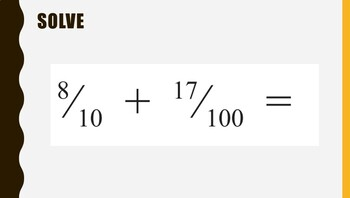# Math - MAFS.4.NF.3.5 - 4.NF.C.5 - 2 Day Power-point Lesson - 10ths & 100thsSubject
Resource Type
File Type

Zip

(394 KB)
Product Rating
Standards
• Product Description
• StandardsNEW

Hello,

This is a power-point lessons that can be thought straight off the smart-board. There are no written plans you would have to do that if your school requires it. At the end of every lesson there is a worksheet that can be used as homework, centers, or pull a few questions for a quick assessment.

MAFS.4.NF.3.5: Express a fraction with denominator 10 as an equivalent fraction with denominator 100, and use this technique to add two fractions with respective denominators 10 and 100. For example, express 3/10 as 30/100, and add 3/10 + 4/100 = 34/100.

LT 5.1: Express a fraction with a denominator of 10 as an equivalent fraction with a denominator of 100. (MAFS.4.NF.3.5)

LT 5.2: Add two fractions with denominators 10 and 100. (MAFS.4.NF.3.5)

Express a fraction with denominator 10 as an equivalent fraction with denominator 100, and use this technique to add two fractions with respective denominators 10 and 100. For example, express 3/10 as 30/100, and add 3/10 + 4/100 = 34/100.
Total Pages
N/A
N/A
Teaching Duration
2 hours
Report this Resource to TpT
Reported resources will be reviewed by our team. Report this resource to let us know if this resource violates TpT’s content guidelines.